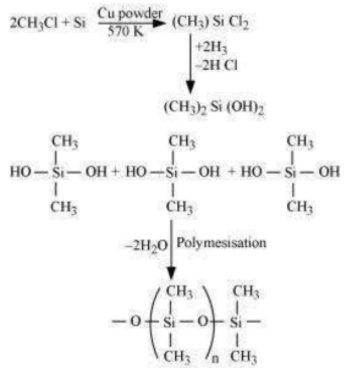Give reasons:

(i) Conc. $HN{O}_{3}$ can be transported in aluminium container.

(ii) A mixture of dilute NaOH and aluminium pieces is used to open drain.

(iii) Graphite is used as lubricant.

(iv) Diamond is used as an abrasive.

(v) Aluminium alloys are used to make aircraft body.

(vi) Aluminium utensils should not be kept in water overnight.

(vii) Aluminium wire is used to make transmission cables.

(a) When silicon reacts with methyl chloride in the presence of copper (catalyst) and at a temperature of about 537 K, a class of organosilicon polymers called methyl-substituted chlorosilanes () are formed.(b) When silicon dioxide ($Si{O}_{2}$) is heated with hydrogen fluoride (HF), it forms silicon tetrafluoride ($Si{F}_{4}$). Usually, the Si–O bond is a strong bond and it resists any attack by halogens and most acids, even at a high temperature. However, it is attacked by HF.
$Si{O}_{2}+4HF\to Si{F}_{4}+2{H}_{2}O$
The SiF4 formed in this reaction can further react with HF to form hydrofluorosilicic acid.
$Si{F}_{4}+2HF\to {H}_{2}Si{F}_{6}$
(c) When CO reacts with ZnO, it reduces ZnO to Zn. CO acts as a reducing agent.
$Zn{O}_{\left(s\right)}+C{O}_{\left(g\right)}\stackrel{∆}{\to }Z{n}_{\left(x\right)}+C{O}_{2\left(g\right)}$
(d) When hydrated alumina is added to sodium hydroxide, the former dissolves in the atter because of the formation of sodium meta-aluminate.